# Hilbert singular integral

The improper integral (in the sense of the Cauchy principal value)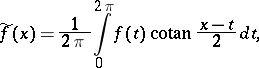where the periodic functionis called the density of the Hilbert singular integral, while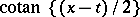is called its kernel. Ifis summable,exists almost-everywhere; ifsatisfies the Lipschitz condition of order,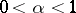,exists for anyand satisfies this condition as well. Ifhas summable-th power,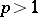,has the same property, and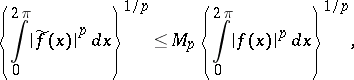where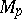is a constant independent of. In addition, the inversion formula of Hilbert's singular integral,is valid. The functionis said to be conjugate with.